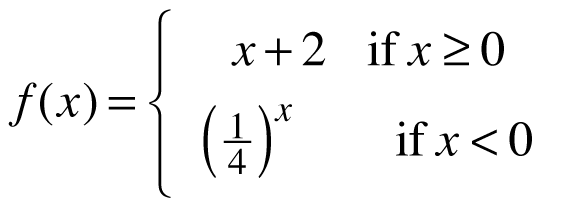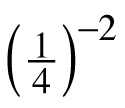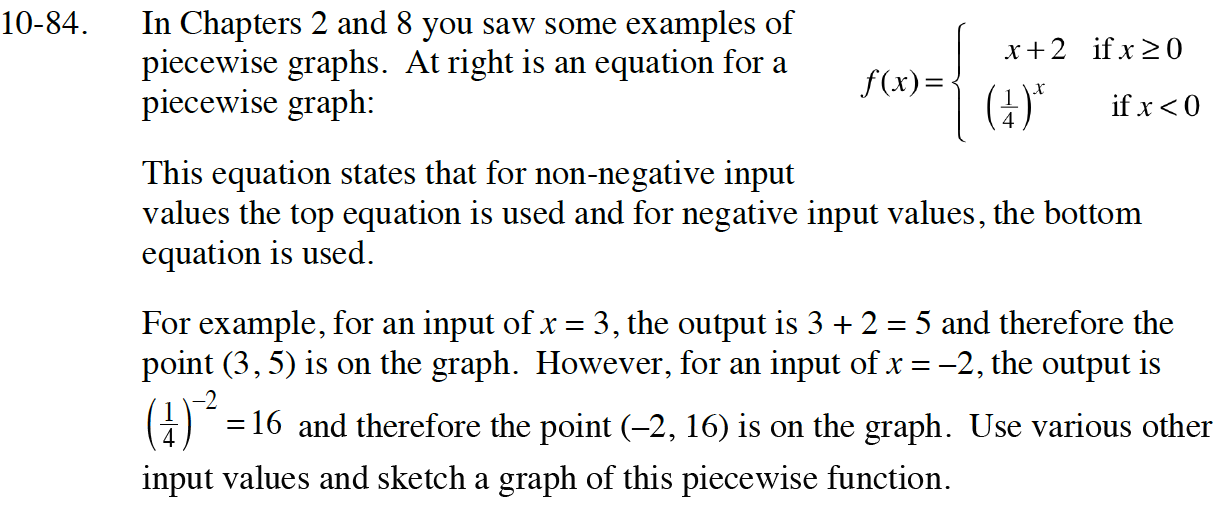### Home > CC4 > Chapter 10 > Lesson 10.2.1 > Problem10-84

10-84.
1.In Chapters 2 and 8 you saw some examples of piecewise graphs. At right is an equation for a piecewise graph: 10-84 HW eTool (Desmos). Homework Help ✎

2. This equation states that for non-negative input values the top equation is used and for negative input values, the bottom equation is used.

3. For example, for an input of x = 3, the output is 3 + 2 = 5 and therefore the point (3, 5) is on the graph. However, for an input of x = –2, the output is= 16 and therefore the point (–2, 16) is on the graph. Use various other input values and sketch a graph of this piecewise function.Complete the table in the eTool below to create a graph of the piecewise function.
Click the link at right for the full version of the eTool: Int1 10-84 HW eTool.